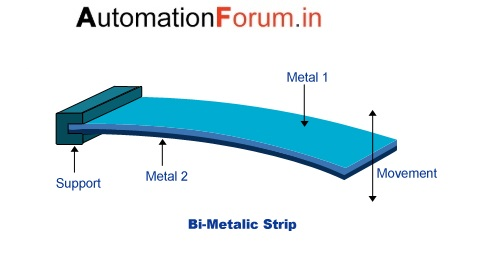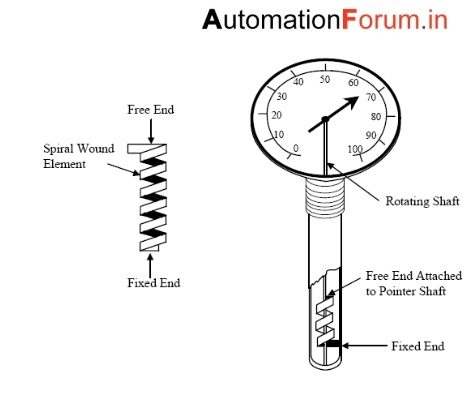# How a Bimetallic thermometer work?

Basic Principle:

These thermometers use the following two principles:

1. All metals change in dimension, which expand or contract when there is a change in temperature.

2. The speed at which this expansion or contraction occurs depends on the coefficient of expansion temperature of the metal and this coefficient of expansion temperature is different for different metals. Therefore, the difference in thermal expansion rates is used to produce deflections that are proportional to changes in temperature.

The bimetallic thermometer consists of a bimetallic strip. A bimetal strip is made of two thin metal strips that have different coefficients of expansion. The two metal strips are joined by brazing, so that the relative movement between them is stopped.

The bimetal strip has the shape of a cantilevered beam. An increase in temperature will result in deflection of the free end of the strip as shown in the diagram. This deviation is linear and may be related to changes in temperature.

The radius of the curvature of the bimetallic strip that was initially planar is determined using the following relationship.

R= t{3(1+m)² + (1+mn)[m²+1/mn]}/6(άh-άl)(T2-T1)(1+m) ² where,

R= radius of the curvature at the temperature T2.

T = total thickness of the bimetallic strip = (t1+t2)

m=t1/t2 = Thickness of lower – expansion metal/thickness of higher – expansion metal.

άl= coefficient of expansion of lower expansion metal.

άh= coefficient of expansion of higher expansion metal.

T1 = Initial temperature.

T2 = temperature.

The following are the important proporties a material should have to be selected for bimetallic thermometers.

1. Coefficient of expansion.

2. Modulus of elasticity.

3. Elastic limit after cold rolling.

4. Electrical conductivity.

5. Ductility.

6. Metallurgical ability.

Different common forms of bimetallic sensors are listed.

1. Helix type.

2. Spiral type.

3. Cantilever type.

4. Flat type.Metals used to make bimetalic thermometer

1. Brass
2. Nickel-iron alloyed with chromium & manganese.
3. Invar (alloy of nickel & iron).

Application

1. The bimetallic strip is used in control devices.

2. The spiral strip is used in air conditioning thermostats.

3. The helix strip is used for process application such as refineries, oil burners, tyre vulcanisers etc.,

Advantages of bimetallic thermometers

1. They are simple, robust and inexpensive.

2. Their accuracy is between +or- 2% to 5% of the scale.

3. They can with stand 50% over range intemperaures.

4. They can be used where evr a mecury –in-glass thermometer is used.

Limitations of bimetallic thermometer:

1. They are not recommended for temperature above 400’C.

2. When regularly used, the bimetallic may permanently deform, which inturn will introduce errors.

Also read

Different methods of temperature measurement